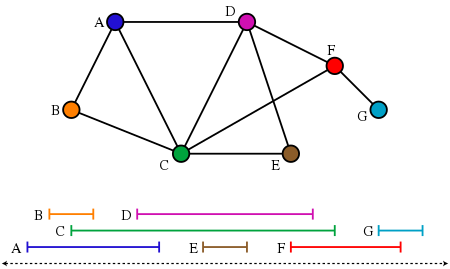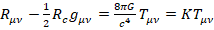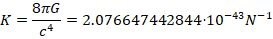## Using Forget in a loop?...

I would like to use the forget command to make maple forget all the things it remembers in each iteration of the loop.

Could someone help me with that?

More details:  I use ansatz to try to solve systems of pdes. sometimes i put the ansatzs in a list and run a loop to try to solve the set of pdes for each ansatz. Sometimes this takes up a lot of memory and maple says kernel connection is lost.

## How to solve large sparse systems of linear equati...

I have some large systems of linear equations.  The solutions are probability generating functions.  I can get solutions in a few minutes for systems of up to n= 200 eqns or so, but Maple just cycles indefinitely if I try to solve much larger systems.  I really only need to perform Gaussian Elimination, as I only need to solve for one of the n solutions.  The matrices are sparse, there are only 3 non-zero entries per row.  I tried to get help from the manuals but I get the impression that sparse solutions are only available for numeric computations.   Doesn't Maple allow for sparse symbolic solutions?  If so, how to do it?

## Error, (in dsolve/numeric/bvp) initial Newton iter...

Hi everyone
how can i overcome this error to solve this ODE ? tnx in advanced.

 > restart
 > U := 1:L := 10:k := 1:Dea := 0.00001:CA0 := 10:Pe := U*L/Dea:Da := k*CA0^2/Dea:
 > Eq1 := diff(CA(x), x, x) - Pe*diff(CA(x), x)/L = Da*L*CA(x)^2/CA0;(1)
 > BCs := CA(0) = CA0, D(CA)(L) = 0(2)
 > ans := dsolve([Eq1, BCs], numeric);
 >

## ShowSolution does not compute the indeterminate li...

In the following I show two functions f and g where ShowSolution(Limit(..)) works for f but not for g. Any idea why?

with(Student[Calculus1]);

f := x^2 + x:
limit(f, x = -infinity);
infinity
ShowSolution(Limit(f, x = -infinity), maxsteps = 100);
// step-by-step solution is produced

g := x^2 + 3*x:
limit(g, x = -infinity);
infinity
ShowSolution(Limit(g, x = -infinity), maxsteps = 100);
Error, (in Student:-Calculus1:-ShowSolution) unable to compute solution steps

Maple is able to change f into an equivalent expression by factoring out x^2, but does not seem to be able to do it with g.

I attach two screenshots that show this curious behaviour.

## The function IsIntervalGraph needs an additional ...

An interval graph is an undirected graph formed from a set of intervals on the real line, with a vertex for each interval and an edge between vertices whose intervals intersect. Recognizing interval graphs  is in linear time.Seven intervals on the real line and the corresponding seven-vertex interval graph.

`IsIntervalGraph(G)` (was introduced in Maple 2022) tests whether the graph G could be expressed as an interval graph for some collection of intervals. If a graph is an interval graph, then the intervals corresponding to its vertices should be given. However,  `IsIntervalGraph`does not provide such an option, which makes it impossible for me to verify the correctness of the results or see more information.

```with(GraphTheory):
G:=Graph({{1,2},{1,3},{1,4}, {4,2},{4,3}});
IsIntervalGraph(G)
```

true

Therefore, an option like the "certificate" option in SageMath needs to be provided.

```g = Graph({1: [2, 3, 4], 4: [2, 3]})
g.show()
g.is_interval()
g.is_interval(certificate=True)```(True, {1: (0, 5), 2: (4, 6), 3: (1, 3), 4: (2, 7)})

I have looked at the source code of `IsIntervalGraph`and it seems to be checking whether the complement graph is comparability. I am not sure if this transformation can still find the corresponding intervals.

```print(IsIntervalGraph)
proc(G::GRAPHLN)::truefalse;
local G2;
G2 := GraphTheory:-GraphComplement(G);
return GraphTheory:-IsComparabilityGraph(G2);
end proc

print(IsComparabilityGraph)
proc (G::GRAPHLN, { transitiveorientation::truefalse := false,

usecached::truefalseFAIL := FAIL }, ` \$`)::truefalse; local

iscomparability, L, A, result, V; A := op(4, G); result :=

FindTransitiveOrientation(A, transitiveorientation); if

result = NULL then false elif transitiveorientation then V

:= op(3, G); true, GraphTheory:-Graph(V, result) else true

end if end proc
```

By the way, can the  "FindTransitiveOrientation "  in the function `IsComparabilityGraph` be used by the user?

## Is it possible to upgrade some external library to...

https://www.maplesoft.com/support/help/Maple/view.aspx?path=copyright lists some external packages used by Maple, but it appears that certain libraries are of outdated (albeit not obsolete) versions. For example, Maple 2023 uses FLINT 2.6.3 (released in 2020), but the newest stable version of FLINT is 2.9.0. Also, Maple 2023 uses Z3 4.5.0 (released in 2016), but the newest stable version of Z3 is 4.12.1. In addition, Maple 2023 uses GCC 10.2.0 (released in 2020), but the newest stable version of GCC is 13.1. Since they are distributed under free licenses, I can download the most recent (or even nightly) release's source code, but how can I replace the old components that Maple uses by the latest ones by myself?

## Impliment of Artificial Neural Network ...

Hello,

Can we impliment Artificial Neural Network for nonlinear coupled ODE equation with boundary conditions.? In maple

I wont seen any post regarding ANN in mapleprime.

## How to access menus when window is maximized?...

There appears to be a bug in Maple 2023.0 (Build ID 1689885)  that does not allow you to access submenus when the window is maximized.  For example, trying to select "Insert --> Execution Group" does not work, nor does any other submenu (the submenu will not display and is not accessible).  The only workaround is to un-maximize the window, select the submenu (for example "Insert --> Execution Group --> Before Cursor"), and then re-maximize the window.  Everything works as it should as long as the window is not maximized.  This is on Microsoft Windows 11 with a 2160p monitor.  Any suggestions on how to fix this are appreciated.

## Solving sets of PDE perturbatively?...

I dont know if this is a well formulated question but if I had a set of PDES that have a constant appearing in them (say alpha), would there be a way to solve for the PDE such that the right had side is not zero but just has higher order order terms (say alpha^3 and higher).

For example consider (the random set of equations)

eq1 := alpha*diff(f(t, r), r,r) + diff(g(t, r), t) + alpha*g(t, r) = 0;

eq2 := alpha*diff(g(t, r), r) + diff(f(t, r), t,t) + f(t, r) = 0;

Is there a way to solve for the system of pde such that the right hand side is only terms of order alpha^3 and higher?

## Error in solve(): 'badly formed input to solve: no...

My end goal is to find the following three expressions:

chi_1 := collect(X_A,[nnu,nnu]);

chi_2 := collect(X_B,[nnu,nnu]);

chi_3 := collect(X_C,[nnu,nnu]);

I expect these three expressions to be linear combinations of random variables nu (nnu) and nu (nnu).

While calling solve(), I encounter this error:

Error, (in assuming) when calling 'SolveTools:-Engine:-Dispatch'. Received: 'badly formed input to solve: not fully algebraic'

What is exactly the issue here? If it can help you answer my doubt, that argmin expression I defined is composed by conditional means and variances which I computed as in here: conditional_distributions_Version1.mw

The two formulas I am trying to implement in Maple are conditional distribution of a multivariate normal distributionAm I already doing any mistake in conditional_distributions_Version1.mw? An alternative interpretation of mine for these two formulas is: conditional_distributions_Version2.mw. Please check the light-blue-highlighted differences in the conditional variance calculation. This alternative interpretation leads to Finding_Chi_Version2.mw, which I also can't solve() (solver stuck in "evaluating") but at least I don't get the error mentioned above...

I am a bit lost to be honest: Is Finding_Chi_Version1 or Finding_Chi_Version2 the correct interpretation?

Thanks!

## Solving 8 non linear equations with 8 unknowns...

Hi

Can anyone help me with this problem, i need maple to solve 8 non linear equations with 8 unknowns. Ive searched the entire internet, and this is what ive scripted so far. However there seems to be a problem with the solve command.

Ive copied the code beneath and posted the maple file aswell

Script:

restart;
g := 9.82;
p := 999.7;
u := 1.307*10^(-3);

F1 := 18 = x__1^2/(2*g) + x__2;

F2 := x__2 = x__3*13/0.1*x__4^2/(2*g) + x__5*35/0.05*x__1^2/(2*g) + x__6*x__4^2/(2*g) + x__7*x__1^2/(2*g);

F3 := 0.1*p*x__4/u = 0.1*p*x__4/u;

F4 := 0.05*p*x__1/u = 0.05*p*x__1/u;

F5 := 1/sqrt(x__3) = -2*log*2.51/(F3*sqrt(x__3));

F6 := 1/sqrt(x__5) = -2*log*2.51/(F4*sqrt(x__5));

F7 := x__4 = 0.05/0.1*x__1;

F8 := x__8 = x__1*Pi*0.05^2/2;

solve({F1, F2, F3, F4, F5, F6, F7, F8}, [x__1, x__2, x__3, x__4, x__5, x__6, x__7, x__8]);
Error, invalid input: solve expects its 1st argument, eqs, to be of type {`and`, `not`, `or`, algebraic, relation(algebraic), ({list, set})({`and`, `not`, `or`, algebraic, relation(algebraic)})}, but received {18 = 0.5091649695e-1*x__1^2+x__2, x__2 = 6.619144604*x__3*x__4^2+35.64154786*x__5*x__1^2+0.5091649695e-1*x__6*x__4^2+0.5091649695e-1*x__7*x__1^2, x__4 = .5000000000*x__1, x__8 = 0.3926990818e-2*x__1, 1/x__3^(1/2) = (-0.6563108934e-4*log/(x__3^(1/2)*x__4) = -0.6563108934e-4*log/(x__3^(1/2)*x__4)), 1/x__5^(1/2) = (-0.1312621786e-3*log/(x__5^(1/2)*x__1) = -0.1312621786e-3*log/(x__5^(1/2)*x__1)), 38244.07039*x__1 = 38244.07039*x__1, 76488.14078*x__4 = 76488.14078*x__4}

## Hardy-Littlewood maximal function of radial funct...

Dear all

I would like to compute Hardy−Littlewood maximal function : we use polar coordinate for a radial function  and then evalaute integral with respect the radius r

Hardy_maximal_function.mw

## Number of minimal dominating sets containing a ver...

The Dominating set defination and Minimal dominating set definations given belowCode to generate the above.

A Function  F such that given the Graph G and vertex v as input the function should return me the number  of minimal dominating sets in the graph G contating the vertex v.

The logic to code seems to be above me Kind help some one please.

As manually doing is not that easy kind help.

I will surely acknowlege,

Kind help

## How do I calculus the K constant in Einstein's equ...

How can I solve Einstein’s equation and calculus of the value of the K constant in Einstein's equation and the value of the tensor stress energy that fits in this equation?